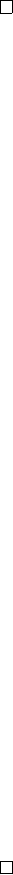5Group actions

IA Groups5.4 Applications
Example.
Let
G
+
be the rotations of a cube acting on the vertices. Let
X
be the set of vertices. Then
|X|
= 8. Since the action is transitive, the
orbit of element is the whole of
X
. The stabilizer of vertex 1 is the set of
rotations through 1 and the diagonally opposite vertex, of which there are 3. So
|G
+
| = | orb(1)|| stab(1)| = 8 · 3 = 24.
Example.
Let
G
be a finite simple group of order greater than 2, and
H G
have index n 6= 1. Then |G| n!/2.
Proof.
Consider the left coset action of
G
on
H
. We get a group homomorphism
ϕ
:
G S
n
since there are
n
cosets of
H
. Since
H 6
=
G
,
ϕ
is non-trivial and
ker ϕ 6
=
G
. Now
ker ϕ C G
. Since
G
is simple,
ker ϕ
=
{e}
. So
G
=
im ϕ S
n
by the isomorphism theorem. So |G| |S
n
| = n!.
We can further refine this by considering
sgn ϕ
:
G
1
}
. The kernel
of this composite is normal in
G
. So
K
=
ker
(
sgn φ
) =
{e}
or
G
. Since
G/K
=
im
(
sgn φ
), we know that
|G|/|K|
= 1 or 2 since
im
(
sgn φ
) has at most
two elements. Hence for
|G| >
2, we cannot have
K
=
{e}
, or else
|G|/|K| >
2.
So we must have
K
=
G
, so
sgn
(
ϕ
(
g
)) = 1 for all
g
and
im ϕ A
n
. So
|G| n!/2
We have seen on Sheet 1 that if
|G|
is even, then
G
has an element of order
2. In fact,
Theorem
(Cauchy’s Theorem)
.
Let
G
be a finite group and prime
p
dividing
|G|
. Then
G
has an element of order
p
(in fact there must be at least
p
1
elements of order p).
It is important to remember that this only holds for prime
p
. For example,
A
4
doesn’t have an element of order 6 even though 6
|
12 =
|A
4
|
. The converse,
however, holds for any number trivially by Lagrange’s theorem.
Proof.
Let
G
and
p
be fixed. Consider
G
p
=
G × G × · · · × G
, the set of
p
-tuples
of G. Let X G
p
be X = {(a
1
, a
2
, · · · , a
p
) G
p
: a
1
a
2
· · · a
p
= e}.
In particular, if an element
b
has order
p
, then (
b, b, · · · , b
)
X
. In fact, if
(b, b, · · · , b) X and b 6= e, then b has order p, since p is prime.
Now let
H
=
hh
:
h
p
=
ei
=
C
p
be a cyclic group of order
p
with generator
h
(This h is not related to G in any way). Let H act on X by “rotation”:
h(a
1
, a
2
, · · · , a
p
) = (a
2
, a
3
, · · · , a
p
, a
1
)
This is an action:
0.
If
a
1
· · · a
p
=
e
, then
a
1
1
=
a
2
· · · a
p
. So
a
2
· · · a
p
a
1
=
a
1
1
a
1
=
e
. So
(a
2
, a
3
, · · · , a
p
, a
1
) X.
1. e acts as an identity by construction
2. The “associativity” condition also works by construction.
As orbits partition
X
, the sum of all orbit sizes must be
|X|
. We know that
|X|
=
|G|
p1
since we can freely choose the first
p
1 entries and the last one
must be the inverse of their product. Since
p
divides
|G|
,
p
also divides
|X|
. We
have
| orb
(
a
1
, · · · , a
p
)
|| stab
H
(
a
1
, · · · , a
p
)
|
=
|H|
=
p
. So all orbits have size 1 or
p
, and they sum to
|X|
=
p×
something. We know that there is one orbit of size
1, namely (
e, e, · · · , e
). So there must be at least
p
1 other orbits of size 1 for
the sum to be divisible by p.
In order to have an orbit of size 1, they must look like
(a, a, · · · , a)
for some
a G, which has order p.